#### Min/Average/Max column Chart

In this Excel charting tutorial, you will create the Min/Average/Max column Charts in Excel. You have to create some data for the same.

## Preparation of min max average data for a chart

Please find below the sales data for a week for six items: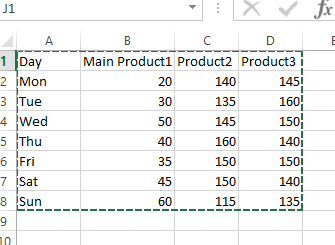Let us insert 3 rows for max, min and avg values: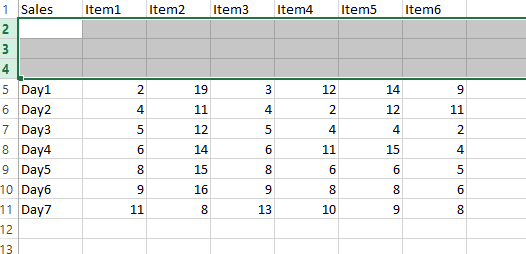Now we have to use formulas to calculate the Max, Min And Average as shown below:

Max: =MAX(B5:B11)

Min: =Min(B5:B11)

Avg: =Average(B5:B11)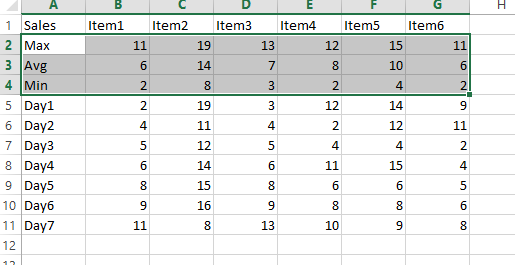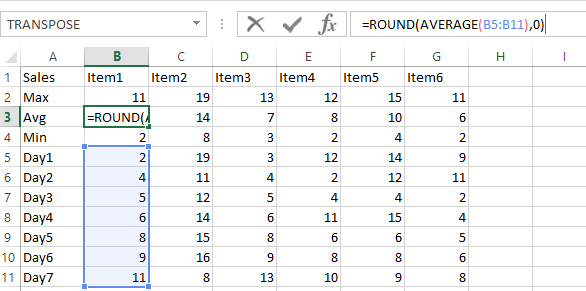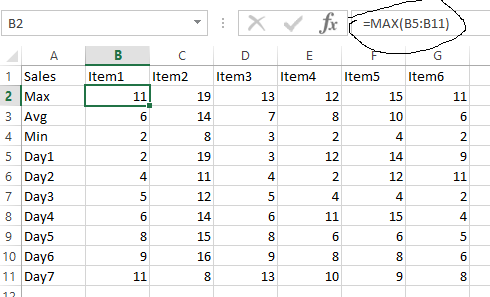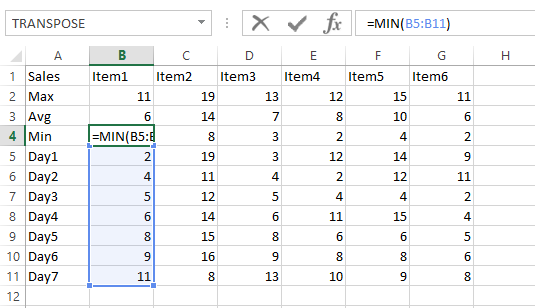## Inserting a min max average chart

Now we have the Max, Min And Avg data for all items. Let’s create the column chart now. Select this data: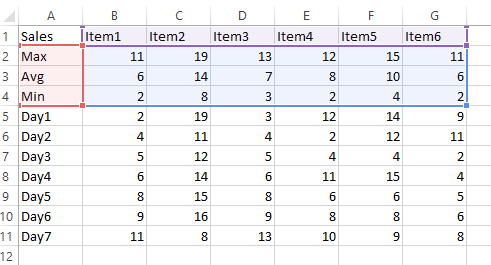Create a 2d column chart: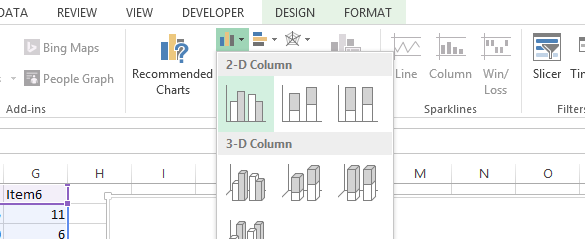It will create the chart as shown below: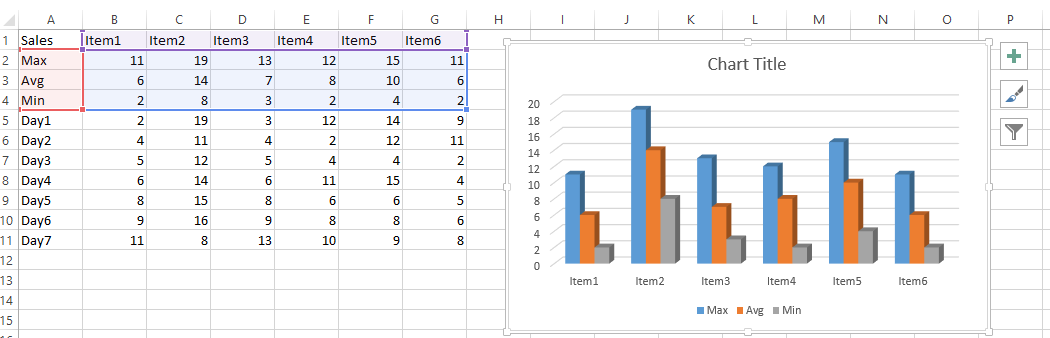So for each item, we have the Max(blue), Min(grey) and Average(Orange) bars as shown.

We can also convert this to a line chart if required as well. Right-click on the chart area and select “change chart type”.By following these steps, you can create a column chart in Excel that displays the minimum, average, and maximum values for a set of data. This can be a useful way to visualize and compare data and draw conclusions from it.